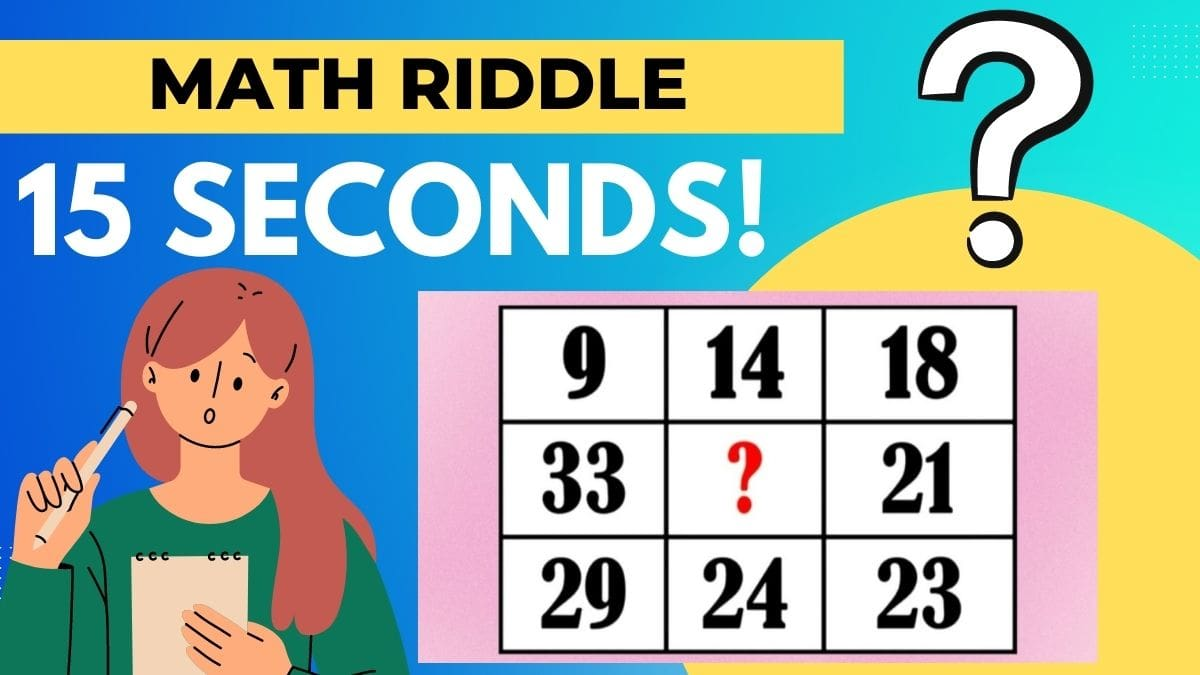# Math Riddles: Can you Solve a Math Table Puzzle In 15 Seconds? Test your IQ here!

Math Riddle Challenge: it's brain exercise time! Can you solve this math table puzzle In 15 Seconds? Test your IQ and sharpen your brain by finding the solution!Math Riddles: Can you Solve Math Table Puzzle In 15 Seconds? Test your IQ here!

Math Riddle Challenge: Solve this interesting math table puzzle. Think about it and solve it! This is what puzzles are about right? identify the problem and answer it in 15 seconds. Only people with high intellectual power can solve it!

What are Math riddles?

Math Riddles are puzzle games where players try to figure out a solution to a problem. As fun as they are, the practice also challenges your brain. They are a great way to boost mental capacity and lateral thinking skills, maintain memory strength, and delight you! It often requires thinking unconventionally with given constraints in mind; sometimes, it involves lateral thinking.

Let’s find out if all of this is true or not! Are you ready?

Here we go!

## Math Riddle Challenge

Look at the picture posted below. You will find a math puzzle that only those with a high IQ will be able to answer. Carefully check this math puzzle and find the missing number.

Is it easy?

Were you able to solve this tricky math puzzle?

If you were able to solve it, let's make it a challenge.

Set a timer to 15 seconds.

Find out how fast you can solve this math puzzle. If you can solve this brain teaser in less time, then that's even better.

Now, go ahead and bring out your mathematical brain to solve this tricky puzzle. Your time starts now.

All the best!

Tick…

Tock…

Tick..

Time’s Up!

 Math Riddle fact! Solving brain teasers regularly can make you brighter and sharper. How? Well, brain teasers are known to improve cognitive functioning and enhance your critical thinking and problem-solving abilities. Also, as a bonus, they help refresh your mind and give you a much-needed break, breaking monotony.

Have you solved the brain teaser yet? We are going to reveal the answers now. Let’s solve it together.

## Solution

To solve a mathematical puzzle, observe the puzzle and try to solve it to find the solution to the problem. If you cannot find the solution, we have an answer for you. If you are still working to figure it out, we have the solution to this mathematical puzzle. This puzzle will allow you to see how keen you are and how reasonable an observer you are. If you still need help, the picture below will help you understand the solution.

### So the answer is 36.

Use mathematical equations and formulas to solve mathematical puzzles. It explains the proper order in which to answer a mathematical question. Many brain puzzles are complex in part because of intuitive human error.

ALSO READ|Math Riddles: Can you Solve This Monkey Puzzle In 15 Seconds? Test your IQ

Math Riddles: Can You Solve This Heart Puzzle In 30 Seconds?

Math Riddles: Do You Have A High IQ? Solve These Figures and Find the Missing Number!

Get the latest General Knowledge and Current Affairs from all over India and world for all competitive exams.
खेलें हर किस्म के रोमांच से भरपूर गेम्स सिर्फ़ जागरण प्ले पर